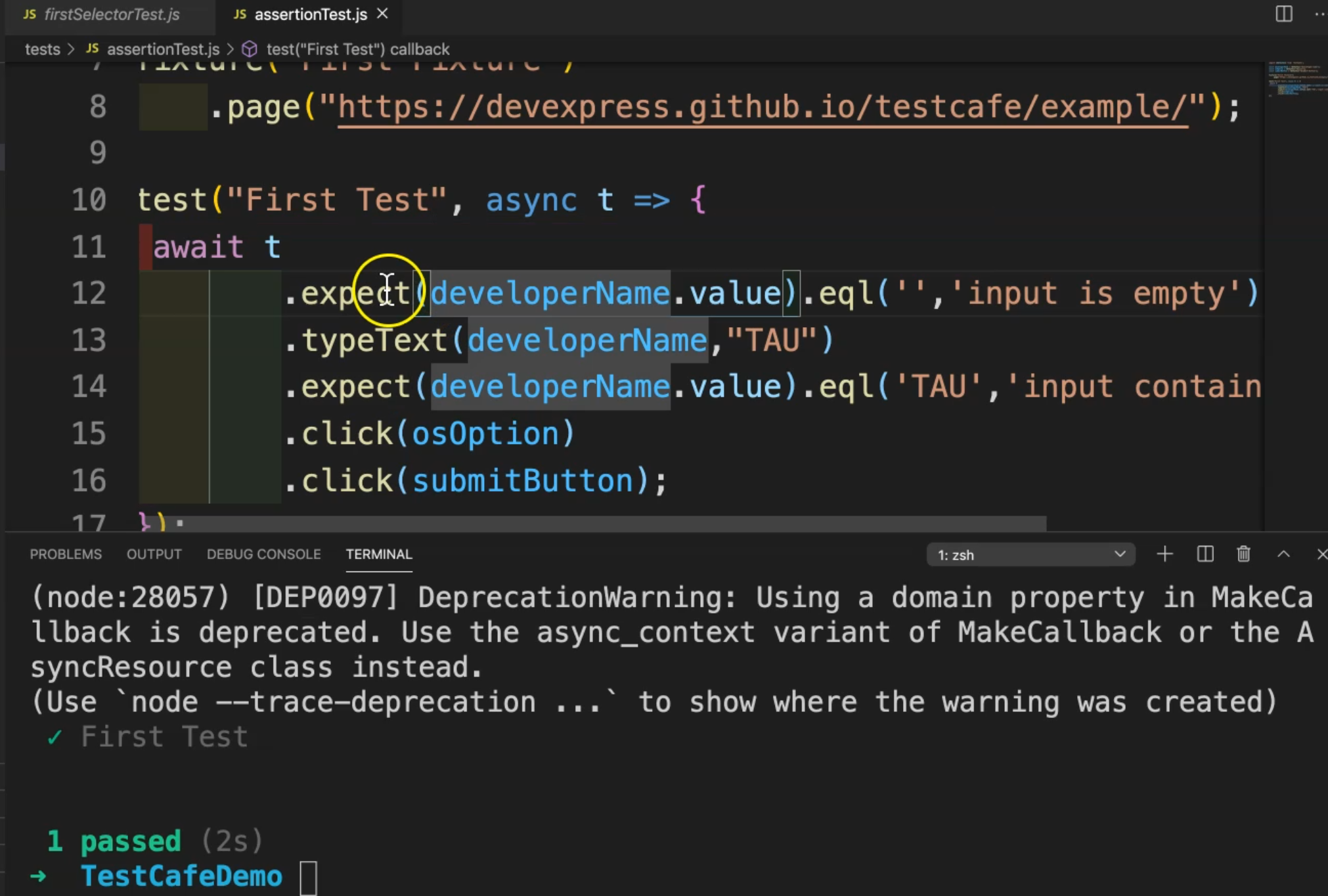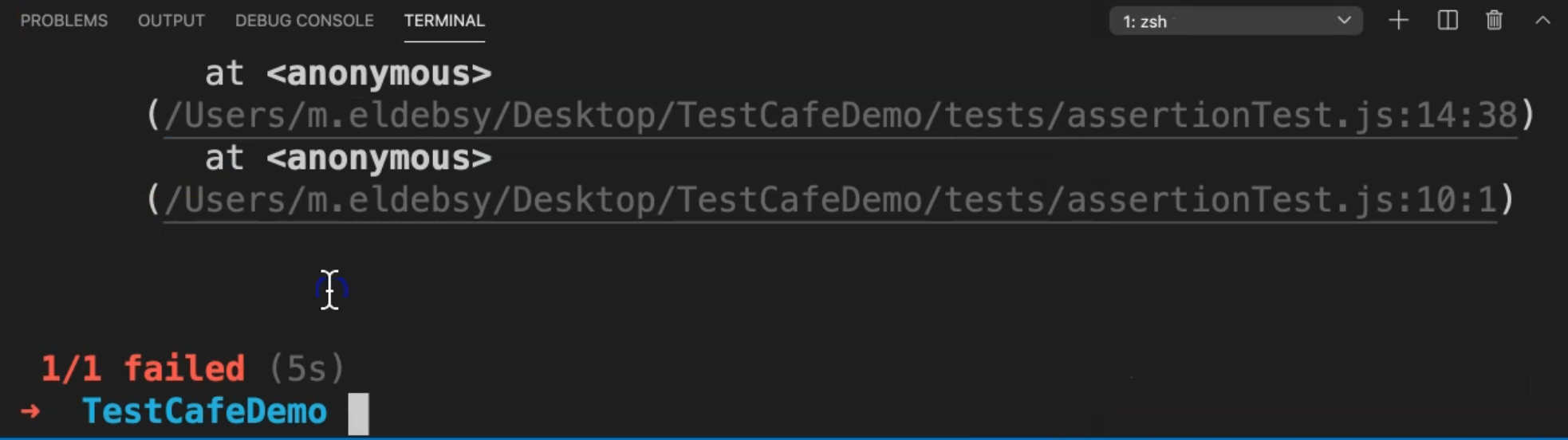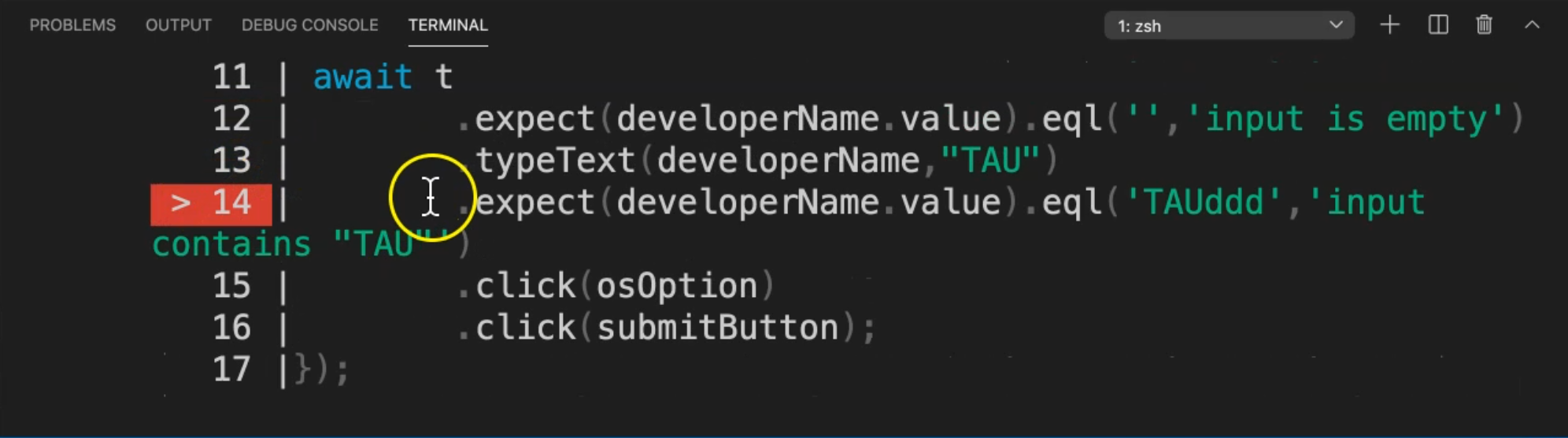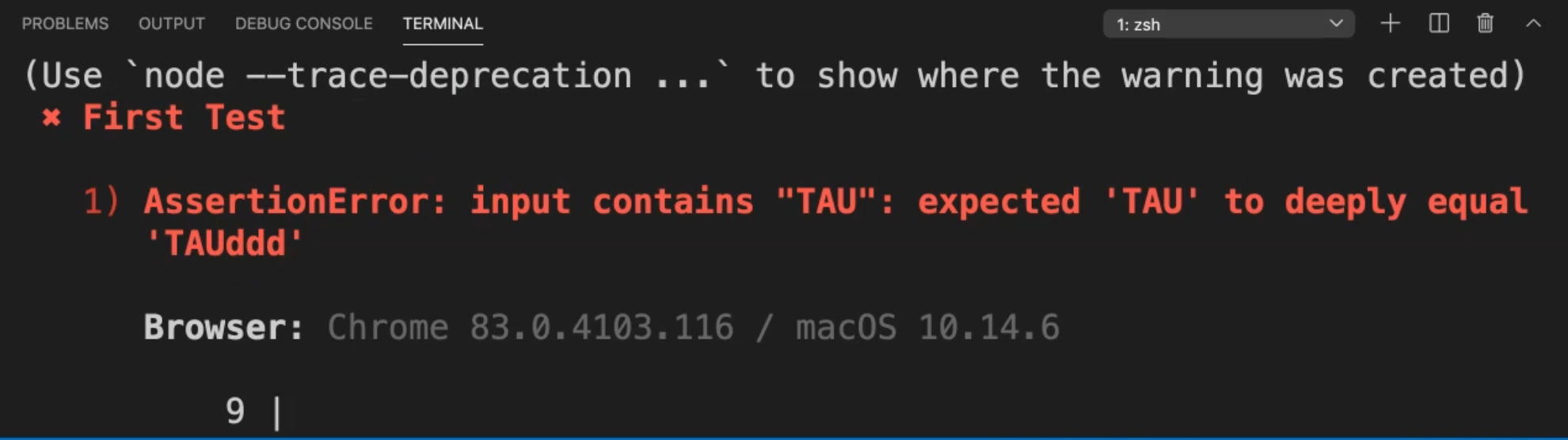Transcripted Summary

We'll be talking about an important topic usually in test automation - assertions.

### # Assertions with TestCafe

TestCafe provides a comprehensive set of assertions that are based on a behavior-driven development style or BDD style.

To construct assertions, use the test controller's expected method.

In this example:

``````await t.expect ( actual ).eql( expected, message, options );
``````

We have `await` with the test controller `t.expect()`. And after that, we add the actual result inside the brackets. Then we need to check that the actual results are equal to the expected one.

`.equal()` takes in parameters `expected`, `message`, and `options` if we wanted to add anything else.

You can specify the assertion query timeout in test code, with the `options.timeout` option.

For example, we need to wait for assertions to be displayed.

So in the example here:

``````await t.expect(Selector('#elementId').innerText).eql('text', 'check element text', { timeout: 500 });
``````

We have `t.expect` - our test controller which expects a Selector. Here we are selecting one Selector from the web application and getting the element's inner text.

We need to assert the inner text inside this element is equal to the text and the message "check element text".

After that, we can pass the time out - for example, 500 milliseconds.

So with assertions, you can only try the assertion, or we can add a timeout with the assertions, with options to timeout.

Before we write our demo, we can check this code snippet to demonstrate how we use assertions in a test.

``````import { Selector } from 'testcafe';

fixture `Example page`
.page `http://devexpress.github.io/testcafe/example`;

test('Check property of element', async t => {
const developerNameInput = Selector('#developer-name');

await t
.expect(developerNameInput.value).eql('', 'input is empty')
.typeText(developerNameInput, 'Peter Parker')
.expect(developerNameInput.value).contains('Peter', 'input contains text "Peter"');
});
``````

For example, we are starting by importing the Selector from TestCafe, and then we add the `fixture` with the example page with the name and then add the page or the URL for the website in our test.

Then, in the `test` that we have 'Check property of element' as the name, and `async` with our test controller `t`.

We will add one constant with `developerNameInput` and the Selector is `#developer-name`. This is the textbox on our website.

Then we need to `expect` that the `developerNameInput` is empty because when we started the application, that textbox was empty at the beginning.

Then `typeText` inside this text box - we will enter this value 'Peter Parker'.

After that, we need to `expect` that the value contains 'Peter', or 'Parker', for example, and the input contains the text 'Peter'.

So this is how we can use - or this is a good snippet for how we can use assertions with TestCafe and in our system.

What are the other methods that TestCafe supports for assertions?

We have different and a huge number of assertions methods with TestCafe like:

• deep equal
• not deep equal
• ok
• not ok
• contains
• not contains
• type of
• not type of
• greater than
• greater than or equal to
• less than or equal to
• within
• not within
• match
• not match

We can use all of them in our tests to verify that the test output is as expected.

### # How to add assertions with TestCafe in our test cases

In this demo, we will learn how to add assertions with TestCafe in our test cases.

We will use the `firstSelectorTest.js` test cases that we used before because we need to use `developerName` and `osOption` and `submitButton` elements in the verification steps with assertions after that.

So here we will copy this file and rename it to `assertionTest.js`.

Then, we need to assert that the `developerName` - or the text box - before we are writing anything inside it, is empty. So here, before `typeText`, we can add something like `.expect()`, which starts an assertion chain that specifies the assertion with the actual value. For the actual value, we need to check that the `developerName` value equals an empty string with `.eql()`. We can set the message to 'input is empty'.

``````import {Selector} from 'testcafe';

const developerName = Selector("#developer-name");
const osOption = Selector("#macos");
const submitButton = Selector("#submit-button");

fixture("First Fixture")
.page("https://devexpress.github.io/testcafe/example/");

test("First Test", async t => {
await t
.expect(developerName.value).eql('','input is empty')
.typeText(developerName,"TAU")
.click(osOption)
.click(submitButton);
});
``````

After we enter the value "TAU", or Test Automation University, we can assert that the `developerName` value is equal - or the input contains "TAU". So again, we can use `.expect()` and check that the `developerName` value equals "TAU" and then the message is "input contains TAU".

``````import {Selector} from 'testcafe';

const developerName = Selector("#developer-name");
const osOption = Selector("#macos");
const submitButton = Selector("#submit-button");

fixture("First Fixture")
.page("https://devexpress.github.io/testcafe/example/");

test("First Test", async t => {
await t
.expect(developerName.value).eql('','input is empty')
.typeText(developerName,"TAU")
.expect(developerName.value).eql('TAU','input contains "TAU"')
.click(osOption)
.click(submitButton);
});
``````

So now we can run our tests:

``````testcafe chrome tests/assertionTest.js
``````

We can check the result. TestCafe is running the test - spinning up a local server, redirecting to the URL to start our test.Here, our test passed.

So after we add the expectations or add our assertions with the `expect` keyword `eql`, the input is empty at the beginning. Then we add value to `developerName`. And after that, we expect that the value is equal to "TAU".

After that, what if we try to change the value of the assertion so it is not equal to the expected one? Then we'll check what happened during our test.

``````import {Selector} from 'testcafe';

const developerName = Selector("#developer-name");
const osOption = Selector("#macos");
const submitButton = Selector("#submit-button");

fixture("First Fixture")
.page("https://devexpress.github.io/testcafe/example/");

test("First Test", async t => {
await t
.expect(developerName.value).eql('','input is empty')
.typeText(developerName,"TAU")
.expect(developerName.value).eql('TAUddd','input contains "TAU"')
.click(osOption)
.click(submitButton);
});
``````

We will run the test again and then check what happened in the step for the verification or assertion.

We run our test, TestCafe will redirect to the URL, we enter the value and here we are waiting for the assertion execution and our test failed.We can scroll up, and then here - this is the failure.The failure always refers to the error line.

Then we can go scroll up further and this is the error - AssertionError: input contains "TAU": expected 'TAU' - but it's finding 'TAUddd' or the wrong value.So we can remove 'ddd' and after that, we can run our test and it will run successfully.

## Resources

Quiz

The quiz for this chapter can be found in 4.10